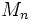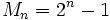# Mersenne number

This article describes a sequence of natural numbers. The parameter for the sequence is a positive integer (or sometimes, nonnegative integer).
View other one-parameter sequences

## Definition

Letbe a natural number. TheMersenne number, denoted, is defined as:.

Sometimes the term Mersenne number is restricted to the case whereitself is a prime number.

Ifitself is prime, it is termed a Mersenne prime. Ifis prime, so is.

## Testing/listing

The ID of the sequence in the Online Encyclopedia of Integer Sequences is A000225
The ID of the sequence in the Online Encyclopedia of Integer Sequences is A001348